## A circular cylinder has a diameter of 3.0 cm and a mass of 25 g. It floats in water with its long axis perpendicular to the water’s surface.

Question

A circular cylinder has a diameter of 3.0 cm and a mass of 25 g. It floats in water with its long axis perpendicular to the water’s surface. It is pushed down into the water by a small distance and released; it then bobs up and down. Part A What is the oscillation frequency

in progress 0
4 weeks 2021-08-17T12:11:57+00:00 1 Answers 1 views 0

f = 5.3 Hz

Explanation:

To solve this problem, let’s find the equation that describes the process, using Newton’s second law

∑ F = ma

where the acceleration is

a =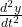B- W = m \frac{d^2 y}{dt^2 }

To solve this problem we create a change in the reference system, we place the zero at the equilibrium point

B = W

In this frame of reference, the variable y’  when it is oscillating is positive and negative, therefore Newton’s equation remains

B’= m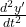the thrust is given by the Archimedes relation

B = ρ_liquid g V_liquid

the volume is

V = π r² y’

we substitute

– ρ_liquid g π r² y’ = m \frac{d^2 y’}{dt^2 }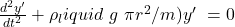this differential equation has a solution of type

y = A cos (wt + Ф)

where

w² = ρ_liquid g π r² /m

angular velocity and frequency are related

w = 2π f

we substitute

4π² f² = ρ_liquid g π r² / m

f =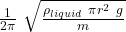calculate

f =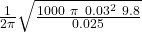f = 5.3 Hz